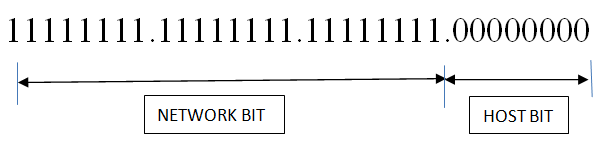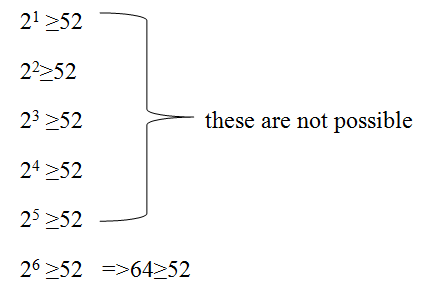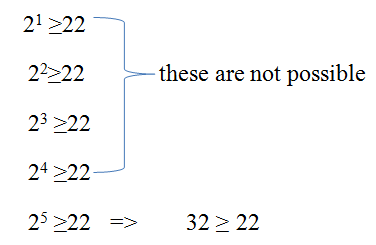# Fixed Length and Variable Length Subnet Mask (FLSM & VLSM)

In this tutorial, we will learn about the fixed length and variable length subnet mask (FLSM & VLSM) in Computer Network. By Anshuman Das Last updated : May 04, 2023

Subnetting means dividing a network in multiple small networks. We use subnetting mainly to save the wastage of IP addresses. We will be discussing here subnetting of classful addressing. There are two types of subnetting,

1. FLSM (Fixed Length Subnet Mask)
2. VLSM (Variable Length Subnet Mask)

We will be discussing here, how to perform subnetting step by step in both cases?

## 1) FLSM (Fixed Length Subnet Mask)

Let’s, consider an IP address 198.168.10.0

Now we have to divide this IP address into 4 subnetwork parts. After dividing we have to find out the range of IP addresses blocks.

4 Simple Steps for Calculating Network Blocks of IP Address

Step 1: Calculate the required subnet bit. In this subnetting process, we will borrow the bit from the host side.

Now here is the question arise, which bits belong to the host side and network side?

We will find it with the help of subnet masks. We are given a Class C IP address and default subnet mask for Class C is 255.255.255.0

Every octet consists of 8-bit in this case we are having 24 1’s in network bit.As we know that every binary value having two possible values either 0 or 1. And with one binary we can make two value and with two binary values, we can make four values. And with three binary value, we can make 8 values and so on.

```    i.e.
21 = 2
22 = 4
23 = 8
24 = 16
```

Here, we need to configure 4 subnets for this we need to borrow 2 bits and making the MSB of host bit of subnet mask to 1 and the value we will get,

11111111.11111111.11111111.11000000

From this we get the mask value for the subnet.

Step 2: In this step we will be calculating updated subnet mask.

i.e. 255.255.255.192 (The decimal equivalent of upper mask in binary)

Step 3: We will find the range in this step.

Formula,

Maximum Subnet Mask is always 255.255.255.255 for every case

```    255.255.255.255
- 255.255.255.192
=   0.  0.  0. 63
```

Here is the range for network we have got is 0.0.0.63

Step 4: Now we will divide the whole network with the help of the range.

First Network Block: 198.168.10.0 – 198.168.10.63

For this block Network ID will be 198.168.10.0 and Broadcast address will be 198.168.10.63

Second Network Block:198.168.10.64 – 198.168.10.127

For this block Network ID will be 198.168.10.64 and Broadcast address will be 198.168.10.127

Third Network Block: 198.168.10.128 – 198.168.10.191

For this block Network ID will be 198.168.10.128 and Broadcast address will be 198.168.10.191

Fourth Network Block:198.168.10.192 – 198.168.10.255

For this block Network ID will be 198.168.10.192 and Broadcast address will be 198.168.10.255

Subnet masks will remain same for every network block i.e. 255.255.255.192

## 2) VLSM(Variable Length Subnet Mask)

Difference between FLSM and VLSM is that VLSM divide Network into multiple networks according to its necessity. In this type of subnetting, you will be given no of required IP addresses and you have to find the Network Blocks accordingly.

Let’s take the same IP address 198.168.10.0,

This time it is given that I have to configure such a network in such a way that given network able to provide 50 IP addresses and 20 IP addresses.

3 Simple Steps for Calculating Networks Blocks

Step 1: Calculate host bit.

For the first case it is given 50 IP addresses is required but 1 IP is required for Network address and another is for Broadcast address. So total requirement is 50+2=50 IP addresses.

For providing that much value we have to compare like this way: if,From here we can get that 6 Host bit is required,
Host bit = 6

Step 2: Find out Network bit with the help of the given formula

Network bit= (32 – Host bit)
In this case Network bit = 32 – 6 =26

Step 3: Calculate updated subnet mask

As we all know network bit is represented by 1 and Host bit is represented by 0.

Now we have the updated subnet mask:

11111111.11111111.11111111.11000000
255.255.255.192

Now, we will find the range using the formula which we have used in FLSM, i.e.
Then we will get the final Range

```    255.255.255.255
- 255.255.255.192
=   0.  0.  0. 63
```

Network Block for the 50 IP addresses is 198.168.10.0 to 198.168.10.63
Again, for 20 IP addresses we have to follow same 3 steps.
Total IP required = 22+2=22

Step 1:Host Bit = 5

Step 2: Now, Network Bit= 32 – 5= 27

Network Bit = 1
Host Bit =0
11111111.11111111.11111111.11100000
255.255.255.224

Range is 0.0.0.31

Network Block for 22 IP addresses is from 198.168.10.64 to 198.168.10.95